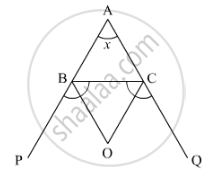Advertisement Remove all ads

# The Bisects of Exterior Angle at B and C of δAbc Meet at O. If ∠A = X°, Then ∠Boc = - Mathematics

MCQ

The bisects of exterior angle at B and C of ΔABC meet at O. If ∠A = x°, then ∠BOC =

#### Options

• $90^\circ + \frac{x^\circ }{2}$
• $90^\circ - \frac{x^\circ }{2}$

• $180^\circ + \frac{x^\circ }{2}$

• $180^\circ - \frac{x^\circ }{2}$

Advertisement Remove all ads

#### Solution

In the given figure, bisects of exterior angles ∠Band ∠C meet at O and  ∠A = x°

We need to find  ext. ∠BOCNow, according to the theorem, “if the sides AB and AC of a ΔABC are produced to P and Qrespectively and the bisectors of  ∠PBC and ∠QCB intersect at O, therefore, we get,

∠BOC = 90^\circ - 1/2 ∠A

Hence, in ΔABC

∠BOC = 90^\circ - 1/2 ∠A

∠BOC = 90^\circ - 1/2 x

Thus,

∠BOC = 90^\circ - x/2

Is there an error in this question or solution?
Advertisement Remove all ads

#### APPEARS IN

RD Sharma Mathematics for Class 9
Chapter 11 Triangle and its Angles
Q 26 | Page 29
Advertisement Remove all ads
Advertisement Remove all ads
Share
Notifications

View all notifications

Forgot password?
Course